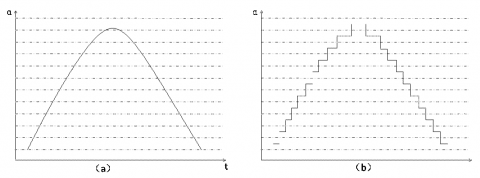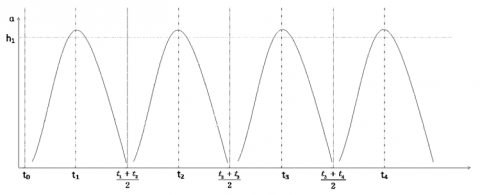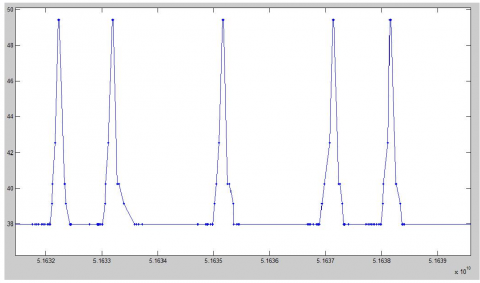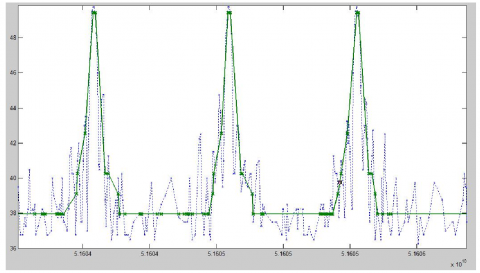# A Novel Frequent Pattern Mining Algorithm for Real-time Radar Data Stream

A Novel Frequent Pattern Mining Algorithm for Real-time Radar Data Stream
Information Science and Engineering College, Shandong Agricultural and Engineering University, Jinan 250100, China

Corresponding Author Email:
qhzbs@21cn.com
Page:
23-30
|
DOI:
https://doi.org/10.18280/ts.360103
29 December 2018
|
Accepted:
22 January 2019
|
Published:
30 April 2019
| Citation

OPEN ACCESS

Abstract:

This paper attempts to improve the radar data quality and discover intrusion behaviors by mining frequent patterns of real-time data. For this purpose, the author improved the frequent pattern mining algorithm for static datasets, and proposed a maximum frequent pattern mining algorithm based on bitmap mapping. Next, a new frequent pattern mining algorithm was designed specifically for data stream with the storage structure of index pattern tree (IPT). The new algorithm was applied to process the real-time radar data stream. The application results demonstrate efficiency of our algorithm in time and space.

Keywords:

frequent pattern, data mining, radar data, data stream, index pattern tree (IPT)

1. Introduction

With the proliferation of information technology, a huge amount of data has been generated in various fields. These data contain both the traditional static data, which are stored in databases, and the real-time and infinite data stream . Typical examples of the data stream include the instantaneous information that is continuously generated in network monitoring, and the various trend charts produced in the stock market. The real-time nature raises higher requirement for the mining of data stream. After all, it is impossible to store all data in the memory, as the data stream is being produced infinitely. If applied to data stream, the frequent pattern mining algorithm will face problems in mining ability and storage structure.

The above problems are particularly severe in the military field. Since WWII, radar has been widely used for military and civil purposes, yielding a massive amount of radar data with complex structure. The shear volume of the data, coupled with the high precision, makes it extremely difficult to find useful information by manual method. Therefore, more and more data mining algorithms, e.g. sequential pattern mining based on association rules , have been introduced to extract features and mine out information from the radar data. Through data analysis and sequential pattern mining, these algorithms manage to excavate the sequence pattern law, enhance the signal quality, predict scanning result, detect intrusions/faults and assist reconnaissance.

In view of the above, this paper improves the frequent pattern mining algorithm and carries out preprocessing based on actual radar data. The improved algorithm was applied to process the real-time radar data stream. The application results demonstrate efficiency of our algorithm in time and space.

2. Literature Review

Since its birth in 1995, the sequential pattern mining  has developed into an important branch of data mining. Apriori-based sequential pattern mining algorithms  like AprioriAll , AprioriSome  have attracted much attention from scholars. For example, Bureva et al.  extended the frequent pattern mining, which extracts frequent item sets into a generalized sequential pattern (GSP) algorithm. Masseglia et al.  proposed the PSP algorithm with prefix tree as the storage structure. Zhang et al.  developed the FFS algorithm to solve the input/output (I/O) consumption problem in the GSP. The above mining algorithms all generate lots of candidate sets, and thus a considerable storage space.

Drawing from the pattern growth, Han et al.  designed the frequent pattern (FP) growth algorithm that generates no candidate set. The same idea was reflected in Hsu’s free-space algorithm  and Pei’s prefix-span algorithm . In addition, Huang et al.  put forward the memory indexing for sequential pattern mining (MEMISP) algorithm based on memory index, which achieves high CPU and memory utilization rate by reading data into the memory and scanning the database only once.

Since 2000, the mining of data stream has gradually attracted the attention from the academia. For instance, Guha et al.  created a novel data stream management system called the STREAM. Zhou et al.  proposed the tilted time window strategy after exploring clustering, classification and frequent pattern mining. Guo et al.  presented an approximation algorithm to mine frequent patterns of time series. Desai et al.  designed the FTP-DS algorithm to mine the frequent patterns of time series. Asai et al.  set up an online mining algorithm called StreamT, which is able to mine frequent ordered trees.

The sliding window model can mine out recently arrived data, without specifying the start and end points of the window. In this model, the processing range of data can be adjusted by sliding on the window. Chang et al.  proposed a frequent pattern mining algorithm based on sliding window model, which mines the frequent patterns of data streams in the current window and saves the mining results in the prefix tree. Chi et al.  invented a heuristic moment algorithm that mines closed frequent patterns by continuous sliding windows in data streams. Inspired by sliding window, Chen et al.  used the storage structure of SWP-tree to mine frequent patterns in data stream through dynamic adjustment of the window size. Lee et al.  put forward the WMFP-SW algorithm to mine frequent patterns in data streams based on the weighted processing of items on the sliding window model; the correctness of the extracted patterns is judged according to the weighted values.

3. Bitmap-Based Improved Maximum Frequent Pattern Mining Algorithm

The bitmap representation refers to mapping the data of sequence database into a bitmap in memory, using binary bits 0 and 1 for storage. In the subsequent analysis, all sequence operations are based on bitmap rows in memory, eliminating the need for second scan of the sequence database.

In this paper, the radar data are inputted in the form of a data stream, which differs from the traditional consumer shopping data for sequential pattern mining. Before mapping, the radar data were denoised and processed in blocks, because the original data are both noisy and infinite. Next, each piece of processed data was mapped to the storage structure according to bitmap mapping, laying the basis for frequent pattern mining.

3.1 Bitmap representation of time series data

The sequence mining was first proposed based on the shopping sequence of consumers. Thus, the data processing often involves the consumer shopping data. In general, the shopping data are saved in the database with joint primary keys like consumer identifier (CID) and transaction identifier (TID). During data preprocessing, the same CID data in the consumer shopping database are merged. The final result (Table 1) will serve as the input of the sequential pattern mining algorithm.

Table 1. Data model of consumer shopping database after merging

 CID Sequence 1 (a, b) a (a, c) 2 aa (a, c) b 3 (a, b) a (ac)

 ID attr1 attr2 ……. t 1 22.6 100 …… 1.11 2 22.7 127 …… 1.12 3 23.8 125 …… 1.13 4 27.6 133 …… 1.15 5 27.8 185 …… 1.17 6 28.1 200 …… 1.19 7 23.5 361 …… 1.23 8 22.9 298 …… 1.26 9 22.5 424 …… 1.29 10 22.3 339 …… 1.36

Unlike consumer shopping data, radar data are stored in the form of data stream in the database (Table 2). Due to the difference in data form, it is impossible to merge and process the radar data by the traditional method. To solve the problem, the data were normalized and data blocks were mapped into the memory, before frequent pattern mining.

As mentioned above, bitmap representation aims to map the data in the database into a bitmap. To compute the support degree effectively, the sequence of item sets in the database was divided into different sets. If its length falls between 2k+1 and 2k+1, the sequence was considered as a sequence of 2k+1 bits, where the minimum value of k is 1. Figure 1 presents the bitmap mapped from the data in Table 1.

## 1.pngFigure 1. Bitmap diagram of sequence database

The sequential database needs to be scanned multiple times in common mining algorithms based on candidate sets, and twice in mining algorithms based on pattern growth. By contrast, the bitmap-based mining only needs to scan the database once, because the sequence information in the database is mapped directly to bitmaps for storage. Therefore, the radar database in this research is mapped into memory, such that all subsequent operations are performed on the bitmap, eliminating the need for a second scan. This perfectly suits the constraint that the radar database can only be scanned once.

After the radar data were denoised and processed in blocks, the data in the current block still cannot be fully mapped into a bitmap and saved in the memory. This calls for a special data structure to store the mapped data. Thus, this paper designs a new algorithm that maps sequential data into linked storage data structure (Figure 2).

## 2.pngFigure 2. Structural diagrams of bitmap mapping for storing data

3.2 Maximum frequent pattern mining algorithm based on bitmap mapping

The proposed algorithm integrates bitmap mapping with recursive division. The frequent sequence patterns are mined out from radar sequence data in such steps as source partitioning, denoising, block processing, bitmap mapping and recursive sequential pattern mining.

Specifically, the proposed algorithm firstly divides the original database into data sources, ensuring that all input sequences are from the same radar. On this basis, the radar database is established. Next, the denoising plan is adopted to smooth the jamming in radar data, followed by the determination of the size of data blocks. After that, the mapping from radar database to bitmap is completed by sequential pattern mining (SPAM) algorithm. Finally, the frequent sequential patterns are mined out from the radar data.

## 3.pngFigure 3. Principle of radar signal noise processing

The radar data were denoised by the data interval division method. Under the rule of sequential pattern representation in Figure 3(a), the values of attribute $α$  were divided into several intervals. The value of each interval was replaced by a specific value. The processed data are plotted as Figure 3(b).

The length of each data interval can be calculated by:

length=(H-L)/n (1)

where, H and L are the maximum and the minimum length of radar data that can be obtained, respectively; n is the number of data intervals. The expression of length can be obtained from formula (1). Then, the mean value of points in each interval can be determined by:

L+(2i-1)/2 length (1≤i≤n) (2)

According to formula (2), it can be calculated that the values of 1 to n intervals are in the order of L+1/2 length, L+3/2 length, …, H-1/2 length, respectively.

As mentioned before, the data in the data stream must be processed in blocks rather than processed all at once, due to the infinite nature of the data stream. To determine the block size, this paper presents a method to partition data blocks based on radar signal periodicity. Assuming that the radar signals have the following periodic regularity, the block processing plan based on the periodic data is shown in Figure 4.

## 4.pngFigure 4. Diagram of radar data block processing

## 5.pngFigure 5. Bitmap mapping of radar signal test data

When the data are mapped from the radar database to memory, a special data structure is needed to store the mapping of original data. The mapping data structure adopted in our algorithm is explained as follows.

Table 3 shows the data of radar data sequence after source partitioning, denoising and block processing.

Table 3. Processed data of radar data sequence

 Sequence ID Sequence 1 a b a c d 2 a b c a c 3 b a b a c 4 a b a c c

After being mapped to the memory by bitmap mapping, the data in Table 3 can be transformed into the form in Figure 5.

Taking the sequence database S as an example, the projection database can be constructed as shown in Figure 6.

## 6.pngFigure 6. Recursive construction of projection database

The pseudocode description of the proposed algorithm is as follows:

Input: data source

Output: frequent pattern sequence

1.Data Source partition by users;

2.Determine the block size with cycle;

3.Map the database to bitmap;

4.Get first column FC of bitmap;

5.For each item in FC

6.if(item.count<min-sup)

remove item from bitmap;

7.End if;

8.Initial project database PD, sequence S=;

9.For each item in FC

DFS-mining (S, PD, item);

10.End for;

4. A New Frequent Pattern Mining Algorithm Based on Index Pattern Tree Storage Structure

In this section, an ordered sequence Moment algorithm for mining closed frequent pattern itemset is proposed. Moment algorithm uses CET storage structure to maintain all node information. Each update operation will traverse the whole tree structure, which makes it difficult to update data. The proposed algorithm improves the storage structure of CET, constructs the nodes in the index pattern tree, and improves the speed of finding nodes in the update process.

4.1 Data storage model based on index tree

The key to sliding window-based frequent pattern mining from data stream lies in the design of the outline structure. As the window moves, new transactions are constantly emerging, and old ones need to be removed. Each transaction can be represented as binary bits. Then, transactions can be added or deleted by moving the project left, during the window sliding. In this section, the transactions are expressed in binary form  and the item location in each basic window is stored in a chain structure, making ordered sequences minable. The bit sequence of items in each window is shown in Table 4.

Table 4. The bit sequence of items in each window

 Window W1 Window W2 Window W3 a 1101 1011 0111 b 0001 0010 0100 c 0111 1111 1111 d 0101 1011 0110 e 1111 1110 1101

During the construction of the bit list of item set, the items were stored in the chain storage structure (Figure 7).

## 7.pngFigure 7. Chain storage structure

## 8.pngFigure 8. IPT structure in W1 window

The mined data were preserved in the IPT [IPT]. Note that only frequent 1-item sets and closed frequent items could be stored in the structure. Figure 8 presents the IPT structure for the data stream in window W1.

4.2 New frequent pattern mining algorithm

During the mining of closed frequent item sets, the improved moment algorithm mainly constructs the IPT and updates the IPT through window sliding. Taking the dataset in Table 4 for example, the frequent patterns can be mined out in the following steps (window size: 4; minimum support: 50 %):

(1) Initial operations:

The dataset of each window is scanned. Next, the bit sequence representation and the location of each item are saved in a chain structure.

(2) IPT construction:

In the initial state, the IPT and root node are empty and the child node is a frequent 1-itemset. Then, each node is processed by bit "and" operation in a right combination way, producing the child node of the node. If it is frequent, the child node is stored in the IPT. Otherwise, it is not saved until no more frequent item is generated.

(3) IPT update:

Deletion outdated transactions: When the window slides, the node $n_i$  pointed by the header pointer is found in the index table according to the item I, and the support degree of the node $n_i$  and its child nodes are updated.

Addition of new transactions: When the window slides, the support degree of each node is updated according to the bit sequence representation of the sliding transaction in the index table. After updating the information of the items in the index table, the pruning operation is performed to prune the nodes with substandard support degree.

(4) Termination condition:

As the window slides, Step (3) is repeated until there is no data inflow.

The new algorithm improves the storage structure of the original moment algorithm, speeds up the search and update, prunes the substandard nodes, and saves the memory space.

5. Experimental Verification

The mining effect of the proposed algorithm was verified through an experiment on multidimensional radar data. The verification covers three aspects: denoising, data partitioning and frequent pattern mining. The frequent patterns were mined by 1D attributes to analyze to pattern periodicity. The selected test dataset of radar data contains over 7,500 data points. The signal data of the selected dimension consist of two columns, namely, the time of radar data generation (microsecond) and the value of $a_k$  attribute of radar data. Figure 9 presents the local simulation diagram of test dataset. Obviously, the radar signals have periodic variations.

## 9.pngFigure 9. The local simulation diagram of radar signal test dataset

5.1 Denoising

The noise jamming of radar data severely impedes sequential pattern mining. The noise must be removed to achieve accurate mining. Through observation, the value of Attribute $a_k$ of radar data was found to fall between 36 and 50. Here, the radar data are divided into 12 intervals for processing. The denoising results are shown in Figure 10 below. The original radar data and the denoised data were compared to disclose the denoising effect.

## 10.pngFigure 10. The local simulation diagram of radar signal after noise processing

## 11.pngFigure 11. Comparison of radar signal noise jamming before and after processing

5.2 Data partitioning

The radar data were processed in blocks by the peak extraction method. The results are shown in Figure 12, where the solid line is the law of radar data and the part between two dashed lines is an input sequence.

## 12.pngFigure 12. Radar signal data blocking

5.3 Maximum frequent pattern mining

The threshold of support in frequent pattern patterns was set to 50%. To control the number of generated frequent sequence patterns, our algorithm only outputs the maximum frequent sequence. Figure 13 shows a frequent sequence pattern in the mining results. Then, the original data and the mining result were simulated in the same graph (Figure 14).

## 13.pngFigure 13. Radar signal mining results

## 14.pngFigure 14. Comparison of radar signal mining results with original data

Next, the proposed algorithm was compared with the traditional moment algorithm in runtime and space consumption at the minimum support of 15 %, 30 %, 45 %, 60 % and 75 %, respectively. The runtimes and space consumptions of the two algorithms are compared in Figures 15 and 16, respectively.

## 15.pngFigure 15. The comparison of running time between the two algorithms

## 16.pngFigure 16. The comparison of space consumption between the two algorithms

As shown in the above figures, the proposed algorithm was more efficient than the traditional moment algorithm; under a low support, our algorithm consumed less space than the traditional one; with the increase of support, however, the space consumption of our algorithm surpassed that of the traditional algorithm. This is because our algorithm needs to map the sequential database into memory, which takes up a certain amount of space.

6. Conclusions

This paper explores the frequent pattern mining for stream data on real-time radar signals. Then, a bitmap-based mining algorithm was proposed for static data and a sliding window-based closed frequent pattern mining algorithm was developed for stream data. Both theoretical analysis and experiment verification confirmed that the proposed stream data mining method can save memory space and achieve efficient operation in the mining of maximum frequent patterns. The future research will try to overcome the conceptual drift in data stream and design a better storage structure for result update and query.

References

 Shin SJ, Lee DS, Lee WS. (2014). CP-tree: An adaptive synopsis structure for compressing frequent itemsets over online data streams. Ieice Information Sciences 278: 559-576. https://doi.org/10.1016/j.ins.2014.03.074

 Mabroukeh NR, Ezeife CI. (2010). A taxonomy of sequential pattern mining algorithms. Acm Computing Surveys 43(1): 1-41. https://doi.org/10.1145/1824795.1824798

 Luo C, Chung SM. (2012). Parallel mining of maximal sequential patterns using multiple samples. Journal of Supercomputing 59(2): 852-881. https://doi.org/10.1007/s11227-010-0476-1

 Balseiro SR, Loiseau I, Ramonet J. (2011). An Ant Colony algorithm hybridized with insertion heuristics for the Time Dependent Vehicle Routing Problem with Time Windows. Computers and Operations Research 38(6): 954-966. https://doi.org/10.1016/j.cor.2010.10.011

 Kuo RJ, Chao CM, Liu CY. (2009). Integration of K-means algorithm and apriorisome algorithm for fuzzy sequential pattern mining. Applied Soft Computing Journal 9(1): 85-93. https://doi.org/10.1016/j.asoc.2008.03.010

 Toivonen H. (2011). Apriori algorithm. Encyclopedia of Machine Learning 39-40. https://doi.org/10.1007/978-0-387-30164-8_27

 Bureva V, Sotirova E, Chountas P. (2015). Generalized net of the process of sequential pattern mining by generalized sequential pattern algorithm (GSP). Advances in Intelligent Systems & Computing 323: 831-838. https://doi.org/10.1007/978-3-319-11310-4_72

 Tiwari S, Kumar L. (2016). Sequential rule mining in M-learning domain. International Journal of Computer Applications 134(3): 23-29. https://doi.org/10.5120/ijca2016907873

 Fournierviger P. (2014). SPMF: A Java open-source pattern mining library. Journal of Machine Learning Research 15(1): 3389-3393.

 Wang J, Han J, Ying L, Tzvetkov P. (2005). TFP: An efficient algorithm for mining top-k frequent closed itemsets. IEEE Transactions on Knowledge & Data Engineering 17(5): 652-664. https://doi.org/10.1109/tkde.2005.81

 Kumar A, Kumar V. (2013). MISP (Modified IncSpan+): Incremental mining of sequential patterns. International Journal of Computer Applications 65(8): 23-31.

 Shyur HJ, Jou C, Chang K. (2013). A data mining approach to discovering reliable sequential patterns. Journal of Systems and Software 86(8): 2196-2203. https://doi.org/10.1016/j.jss.2013.03.105

 Huang CK. (2009). Developing an efficient knowledge discovering model for mining fuzzy multi-level sequential patterns in sequence databases. Fuzzy Sets & Systems 160(23): 3359-3381. https://doi.org/10.1016/j.fss.2009.06.003

 Lammersen C, Schmidt M, Sohler C. (2015). Probabilistick-Median clustering in data streams. Theory of Computing Systems 56(1): 251-290. https://doi.org/10.1007/978-3-642-38016-7_7

 Zhou Q, Wu TJ. (2007). A recent-biased pattern matching algorithm for time series based on tilted-time window. Information & Control 36(6): 678-666. https://doi.org/10.1016/S1874-8651(08)60023-X

 Nie GL, Lu ZD. (2006). Dynamically computing approximate frequency counts in sliding window over data stream. Wuhan University Journal of Natural Sciences 11(1): 283-288. https://doi.org/10.1007/bf02831748

 Desai D, Joshi A. (2015). A deviant load shedding system for data stream mining. Procedia Computer Science 45: 118-126. https://doi.org/10.1016/j.procs.2015.03.103

 Bifet A, Gavald R. (2011). Mining frequent closed trees in evolving data streams. Intelligent Data Analysis 15(1): 591-599. https://doi.org/10.3233/IDA-2010-0454

 Wang L, Feng L, Jin B. (2014). Sliding window-based frequent itemsets mining over data streams using tail pointer table. International Journal of Computational Intelligence Systems 7(1): 25-36. https://doi.org/10.1080/18756891.2013.859860

 Shin SJ, Lee DS, Lee WS. (2014). CP-tree: An adaptive synopsis structure for compressing frequent itemsets over online data streams. Information Sciences 278: 559-576. https://doi.org/10.1016/j.ins.2014.03.074

 Chen H, Shu LC, Xia J, Deng Q. (2012). Mining frequent patterns in a varying-size sliding window of online transactional data streams. Information Sciences 215(23): 265-294. https://doi.org/10.1016/j.ins.2012.05.007

 Lee G, Yun U, Ryu KH. (2014). Sliding window based weighted maximal frequent pattern mining over data streams. Expert Systems with Applications 41(2): 694-708. https://doi.org/10.1016/j.eswa.2013.07.094

 Sun G, Bin S. (2017). Router-level internet topology evolution model based on multi-subnet composited complex network model. Journal of Internet Technology 18(6): 1275-1283.

 Sun G, Bin S. (2018). A new opinion leaders detecting algorithm in multi-relationship online social networks. Multimedia Tools and Applications 77(4): 4295-4307.